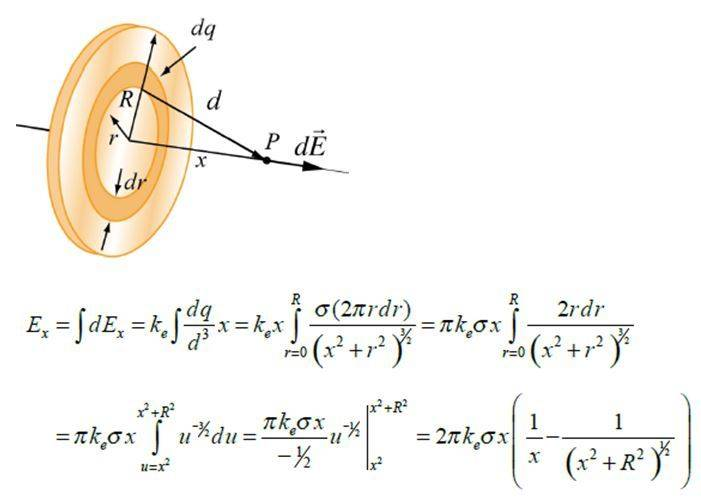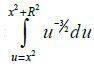# Electric field of a disk & u-substitution

## Homework Statement

Calculate the electric field at point P (refer to visual):## Homework Equations

I follow everything - except how the limits of integration for the u-integral () are arrived at?

## The Attempt at a Solution

u=x2 is the smallest value u can have, and u=x2+R2 is the greatest value u can have?